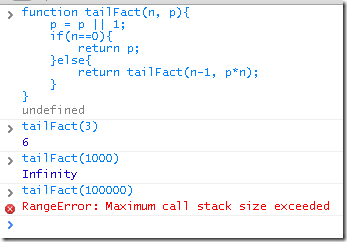`function fact(n)    return n==0 and 1 or n * fact(n-1)end`

`function tail_fact(n, p)    p = p or 1    if n==0 then        return p    end    return tail_fact(n-1, n*p)end`

http://en.wikipedia.org/wiki/Tail_call

Lua是支持尾递归的，所以上面的尾递归的代码，是可以很好的工作的。

### Python的尾递归支持

Python本身是不支持尾递归的(via)，并且对递归次数有限制的，当递归次数超过1000次的时候，就会抛出“RuntimeError: maximum recursion depth exceeded”异常。

`#recursion.py@tail_call_optimizeddef fact(n):    return 1 if n==0 else n * fact(n-1)`

`#tail_recursion.py@tail_call_optimizeddef tail_fact(n, p=1):    if n==0:        return p    return tail_fact(n-1, n*p)`

### Node.js对尾递归的支持

• “然而不幸的是。目前已知的javascript 的解释环境中并不支持这种特性（尾递归）。因此，我们在这里讨论函数室式时，可以说“能够通过函数递归来消灭循环语句”，但在不支持尾递归（及其优化技术）的javascript中，这种实现将以大量栈和内存消耗为代价”`// recursion.jsfunction fact(n){    if(n == 0){        console.log('fact: ');        console.dir(process.memoryUsage() );    }    return n==0 ? 1 : n * fact(n-1);}`

`// tail_recursion.jsfunction tailFact(n, p){    p = p || 1;    if(n==0){        console.log('tail fact: ');        console.dir(process.memoryUsage() );        return p;    }else{        return tailFact(n-1, p*n);    }}`

`\$ node recursion.js fact: { rss: 7892992,  vsize: 55169024,  heapTotal: 2861216,  heapUsed: 1634612 }`

`\$ node tail_recursion.js tail fact: { rss: 7901184,  vsize: 55169024,  heapTotal: 2869376,  heapUsed: 1791520 }`

`var start = Date.now();for(var i=0; i<1000; i++){    tailFact(13000);}console.log( (Date.now() - start)/1000 );`

`\$ node recursion.js 0.257\$ node tail_recursion.js 0.277`

Enjoy!